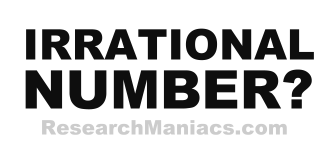Is 8 an Irrational Number?The number 8 is an irrational number if 8 canNOT be expressed as a ratio, as in irRATIOnal.

A quotient is the result you get when you divide one number by another number. For 8 to be an irrational number, the quotient of two integers canNOT equal 8.

In other words, for 8 to be an irrational number, 8 canNOT be expressed as a ratio where both the numerator and denominator are integers (whole numbers).

The number 8 can be expressed as 8/1 where 8 is the numerator and 1 is denominator.

Thus, the answer to the question "Is 8 an irrational number?" is NO.

Irrational Number?
Enter another number to see if it is irrational:

Is 9 an Irrational Number?
See information about the next number on our list.